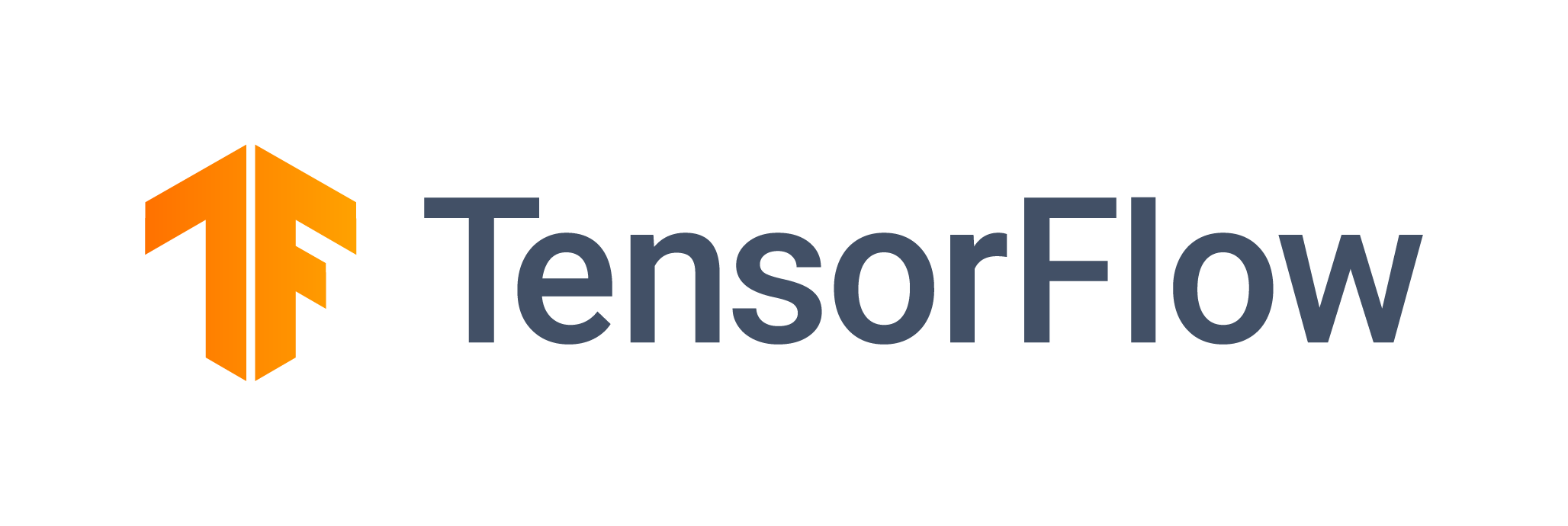# Train a TensorFlow Model (Multi-GPU)

Connect multiple GPUs to quickly train a TensorFlow model## Overview

This example builds on Single-Node Single GPU Training in TensorFlow. It trains a Resnet50 model on a dataset of bird images to identify different species of birds. In this example, we will be using multiple GPUs. We will parallelize the learning by using TensorFlow Mirrored Strategy. The model will split the data in each batch (sometimes called a “global batch”) across the GPUs, thereby making “worker batches.” Each GPU has a copy of the model, called a “replica,” and after they learn on different parts of each batch, they will combine the learned gradients at the end of the step. The result at the end of training is one model that has learned on all the data.

This dataset consists of 40,000+ images of birds and has been taken from kaggle.

We recommend spinning up a bigger Saturn Cloud instance now, with multiple GPUs, so we can distribute the training work and have the GPUs work simultaneously, all training the same model.

``````import tensorflow as tf
import keras
import time
import matplotlib.pyplot as plt
``````

The dataset originally had 285 classes. We have taken a subset of this data, which has 61 classes. The data is stored in AWS S3. The first time you run this job, you’ll need to download the training and test data, which will be saved in `dataset/birds/`.

``````import s3fs

s3 = s3fs.S3FileSystem(anon=True)
_ = s3.get(
rpath="s3://saturn-public-data/100-bird-species/100-bird-species/*/*/*.jpg",
lpath="dataset/birds/",
)
``````

Our dataset has already neatly separated training, test, and validation samples. In the code below, we construct Keras data objects for training and validation sets using `keras.preprocessing.image_dataset_from_directory`. We chose the Adam optimizer and set the learning rate to 0.02. We train our classifier with ResNet50 model architecture, which has 48 Convolution layers along with 1 MaxPool and 1 Average Pool layer. The model is compiled, trained, and saved in `model/keras_single/`. This function is actually incredibly similar than our single GPU approach. We’re simply applying the Mirrored Strategy scope around our model definition and compilation stage, so that TensorFlow knows this model is to be trained on multiple GPUs.

``````def train_multigpu(n_epochs, classes, base_lr, batchsize, scale_batch=False, scale_lr=False):

strategy = tf.distribute.MirroredStrategy()
print("Number of devices: %d" % strategy.num_replicas_in_sync)

with strategy.scope():
model = tf.keras.applications.ResNet50(include_top=True, weights=None, classes=classes)

model.compile(
loss="sparse_categorical_crossentropy", optimizer=optimizer, metrics=["accuracy"]
)

# Data
train_ds = (
tf.keras.preprocessing.image_dataset_from_directory(
"dataset/birds/train", image_size=(224, 224), batch_size=batchsize
)
.prefetch(2)
.cache()
.shuffle(1000)
)

# printing sample birds images
for birds, labels in train_ds.take(1):
plt.figure(figsize=(18, 18))
for i in range(9):
plt.subplot(3, 3, i + 1)
plt.imshow(birds[i].numpy().astype("uint8"))
plt.axis("off")
plt.show()

valid_ds = tf.keras.preprocessing.image_dataset_from_directory(
"dataset/birds/valid", image_size=(224, 224), batch_size=batchsize
).prefetch(2)

start = time.time()

model.fit(
train_ds,
epochs=n_epochs,
validation_data=valid_ds,
)

end = time.time() - start
print("model training time", end)

tf.keras.models.save_model(model, "model/keras_multi/")
``````

In the code below, we set up necessary parameters. We run only a few epochs to save time. But once you’ve got this model working, you’ll have all the information you need to build and run bigger TensorFlow models on Saturn Cloud. If required, increase the batch size to take full advantage of multi-GPU processing power so that GPUs can be kept busy (but without running out of RAM).

``````model_params = {
"n_epochs": 3,
"base_lr": 0.02,
"batchsize": 64,
"classes": 285,
"scale_batch": True,
}
``````

The code below runs the model training process and saves your trained model object to the Jupyter instance memory. A folder called `model` will be created and populated for you.

``````tester_plain = train_multigpu(**model_params)
``````
``````import tensorflow as tf
import keras
import time
import matplotlib.pyplot as plt

import s3fs

s3 = s3fs.S3FileSystem(anon=True)
_ = s3.get(
rpath="s3://saturn-public-data/100-bird-species/100-bird-species/*/*/*.jpg",
lpath="dataset/birds/",
)

def train_multigpu(n_epochs, classes, base_lr, batchsize, scale_batch=False, scale_lr=False):

strategy = tf.distribute.MirroredStrategy()
print("Number of devices: %d" % strategy.num_replicas_in_sync)

with strategy.scope():
model = tf.keras.applications.ResNet50(include_top=True, weights=None, classes=classes)

model.compile(
loss="sparse_categorical_crossentropy", optimizer=optimizer, metrics=["accuracy"]
)

# Data
train_ds = (
tf.keras.preprocessing.image_dataset_from_directory(
"dataset/birds/train", image_size=(224, 224), batch_size=batchsize
)
.prefetch(2)
.cache()
.shuffle(1000)
)

# printing sample birds images
for birds, labels in train_ds.take(1):
plt.figure(figsize=(18, 18))
for i in range(9):
plt.subplot(3, 3, i + 1)
plt.imshow(birds[i].numpy().astype("uint8"))
plt.axis("off")
plt.show()

valid_ds = tf.keras.preprocessing.image_dataset_from_directory(
"dataset/birds/valid", image_size=(224, 224), batch_size=batchsize
).prefetch(2)

start = time.time()

model.fit(
train_ds,
epochs=n_epochs,
validation_data=valid_ds,
)

end = time.time() - start
print("model training time", end)

tf.keras.models.save_model(model, "model/keras_multi/")

model_params = {
"n_epochs": 3,
"base_lr": 0.02,
"batchsize": 64,
"classes": 285,
"scale_batch": True,
}

tester_plain = train_multigpu(**model_params)

``````